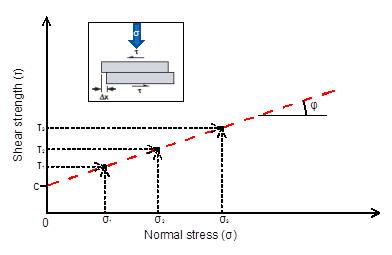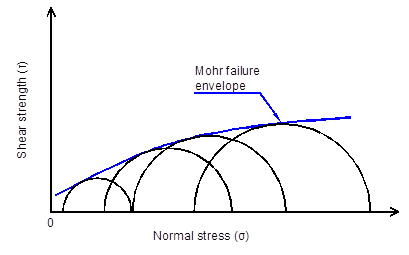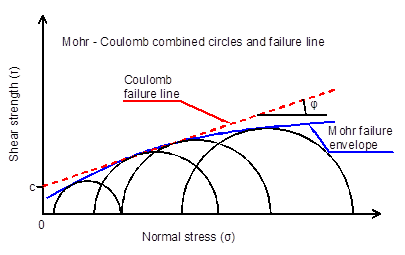# The Mohr – Coulomb strength criterion

This is something that all geotechnical engineers should know but it is surprising how many do not! Just a brief overview of how the Mohr – Coulomb strength criterion came about.

The Mohr – Coulomb criterion is the outcome of inspiration of two great men, Otto Mohr born on 1835 and passed away on 1918 and Charles-Augustin de Coulomb born on 1736 and passed away on 1806.

The two men never coexisted but their brilliant minds contributed significantly in the scientific knowledge. The combination of two hypotheses gave us the Mohr – Coulomb failure surface.

Chronologically,  Coulomb was involved in military defense works (how much knowledge have we gained due to war!) trying to built higher walls for the French. In order to investigate why taller walls than usual were failing and try to built them to stand, he wanted to understand the lateral earth pressure against retaining walls and the shear strength of soils. He devised a shear strength test and observed (at that time, with his tests) that soil shear strength was composed of one parameter that was stress – independent named cohesion (c) and one that was stress – dependent, similar to friction of sliding solid bodies named angle of internal friction (φ). Probably he executed shear strength tests and found for different normal stresses (σ) different shear stresses (τ). By plotting these data on a (τ-σ) diagram he obtained the straight line denoted by the equation τ=c+σ.tan(φ) as can be seen in the next figure.Mohr (1900) proposed a criterion for the failure of materials on a plane which has a unique function with the normal stress on that plane of failure. The equation for that was τ=f(σ) where τ is the shear strength and σ the normal stress on the plane.  With the use of the Mohr circles which is a two dimensional graphical representation of the state of stress at a point and the circumference of the circle is the locus of points that represent the state of stress on individual planes the Mohr failure envelope was proposed. The Mohr envelope was a line tangent to the maximum possible circles at different stresses and no circle could have part of it above that tangent curved line. (figure 2).It is not known (Holtz et al, 1981) who first combined both theories but combining the Mohr failure criterion with the Coulomb equation gave a straight line tangent (to most of the Mohr circles) and the Mohr – Coulomb strength criterion was born (figure 3).Holtz R. D., Kovacs W. D., (1981). “An Introduction to Geotechnical Engineering”, Prentice Hall.